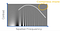# How JPEG Encoding Works?

JPEG stands for “Joint Photographic Expert Group” and it is a lossy data compression algorithm used to compress digital images. It is good at compressing natural photographs but falls short while compressing vector artworks. In this article we’ll see technical details on how this famous encoding algorithm works.

Why should we compress images? — The simple answer is “to save the space”. Consider an example of 3000X4000 pixel image in RGB color space. If each channel of RGB is 8-bit, the size of this image would be 36MB !

But JPEG makes a profitable trade where it reduces the file size (36 MB becomes 2 MB) while maintaining the perceivable image quality. It’s because the thrown-out parts are those parts of the image that are not perceived easily by human eye.

# Encoding Algorithm

## Step 1: Color Space Conversion

In first step let us convert given RBG image into YCbCr color space. This step provides makes groundwork for next step.

## Step 2: Chroma Subsampling

Human eye is more sensitive towards brightness than color, hence we can safely down-sample chroma components to save some space. This strategy is called “Chroma subsampling” and it is used in many image & video processing pipelines.

## Step 3: DCT (Discrete Cosine Transform)

Let’s utilizes another nature of human eye i.e. “Frequency-dependent contrast sensitivity”. In below diagram, we can see that the bars under the curve are more visible than the rest. This gives us some room for compression in those less visible high frequencies in the upper right corner.Look at upper right corner- this is the area where we can perform extreme compression

Now, lets divide the image into 8x8 blocks and take this image data to frequency-domain representation.

How do we do this? We perform DCT in each 8X8 block that we’ve got above. Since Cosine wave varies between -1 to 1 between PI and 2PI, we’ll extrapolate each pixel value to come within the range of [-128, 127) since we are dealing with 8-bit color components here and full range is [0, 255). We call this shifted-block.

Next step is to compare each 8x8 shifted block with 64 frequency patterns.

We basically pick one frequency pattern and see what is its contribution in whole 8x8 shifted block and the resultant is produced in the range of [-1024, 1024). This process decomposes the image into its frequency components, converting an 8x8 block to its frequency counterpart.

In this frequency representation we can easily compress the frequencies which are less visible to us (i.e. upper left corner) by dividing these numbers with some constants and then quantizing them (next step).

## Step 4: Quantization

It simply means rounding these results to nearest integers. If we divide by larger values, more zeros will be obtained but it’ll also man lowering the image quality. After quantization we end up with a lot of zeros in higher frequencies. The higher frequencies that we are less sensitive to get divided by larger constants as compared to the ones that we are more sensitive to.

## Step 5: Run Length Encoding

As we saw above, after quantization, we end up with lot of zeros in high frequencies. We can store this information more efficiently by rearranging these in zig-zag order from top-left to bottom-right. This way we can group the zeros together and we can store them as range like this:

## Step 6: Huffman (entropy) Encoding

We can further compress what’s left by encoding the more frequent values with fewer bits and less frequent values with more bits — a concept used in Huffman Encoding to avoid ambiguity. Please see this story to know all about greedy Huffman Encoding.

Note: Both Run Length and Huffman encodings are lossless methods.

# Decoding Algorithm

While decoding, all of the above steps are reversed:

# Drawbacks of JPEG

JPEG is not recommended to compress vector artworks. It results in lot of jagged edges in those cases, as evident in below diagram. For vector artworks, we can use PNG, WebP or SVG (scalable vector graphics) formats.

# Conclusion

I hope above article helped in demystifying the internal technical steps involved in JPEG compression & decompression.

Written by